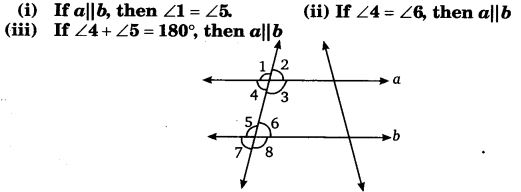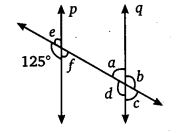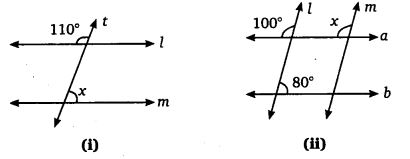# Class 7 Maths NCERT Solutions for Chapter – 5 Lines and Angles EX – 5.2

## Lines and Angles

Question 1.
State the property that is used in each of the following statements?Solution:
(i) Corresponding angle property.
(ii) Alternate interior angle property.
(iii) Interior angles on the same side of the transversal are supplementary.

Question 2.
In the adjoining figure, identify :

1. the pairs of corresponding angles.
2. the pairs of alternate interior angles.
3. the pairs of interior angles on the same side of the transversal.
4. the vertically opposite angles

Solution:

1. ∠1, ∠5; ∠2, ∠6; ∠3, ∠7 and ∠4, ∠8 are four pairs of corresponding angles.
2. ∠2, ∠8 and ∠3, ∠5 are two pairs of alternate interior angles.
3. ∠2, ∠5 and ∠3, ∠8 are two pairs of interior angles on the same side of the transversal.
4. ∠1, ∠3; ∠2, ∠4; ∠5, ∠7 and ∠6, ∠8 are four pairs of vertically opposite angles.

Question 3.
In the adjoining figure p || q. Find the unknown anglesSolution:

Question 4.
Find the value of x in each of the following figures if l || m :Solution:
(i) Since, l || m and t is a transversal.
∴ ∠x = (180° – 110°) = 70° [Corresponding angles, Linear pair]

(ii) if l || m and a is a transversal.
Then, ∠x = 1000 [Corresponding angles]

Question 5.
In the given figure, the arms of two angles are parallel. If ∠ABC = 70°, then find(i) DGC
(ii) DEF

Solution:

Question 6.
In the given figures below, decide whether l is parallel to m.Solution: